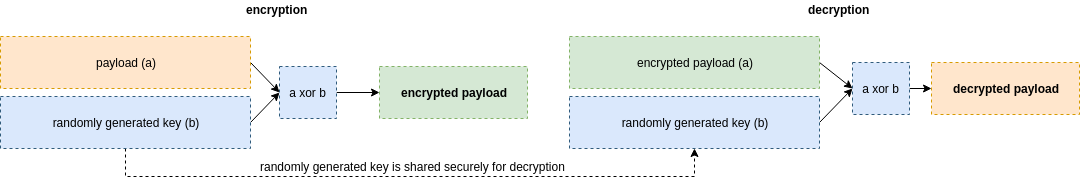# Custom encrypter - One-time pad (OTP)

This blog post has been created for completing the requirements of the SecurityTube Linux Assembly Expert Certification. The task for 7/7 assignment is to create a custom crypter using any existing encryption schema. It can be written in any programming language of one’s choice.

Student ID: SLAE64 - 1594

### Custom encrypter - one time pad

The encryption routine I chose for this assignment is the One-time pad (OTP), which for it to work, needs a pre-shared key. The need for a pre-shared key in order to decrypt the payload is unfortunately one of the problems coming up when talking about the OTP. If the key sharing is not done securely, there is no point of using one-time pads in the first place. As this write-up won’t touch the topic of key sharing one bit, I assume one should come up with idea to share it securely outside the bounds of this little article.

The simple idea behind using a one-time pad can be described with a small example. If we have plain text a, and we have securely pre-shared a secret key b, then to have an encrypted text we would xor a and b. In order to decrypt the message we would use the pre-shared key b again to xor it with the encrypted text instead. In such a way we would end up again with the original text a. The following scheme describes the exact same steps taken:#### Random key generation

How do you create a key that is truely random? This is another problem aside from sharing the key itself. This is the moment, where if you would start looking around in the Internet, you would stumble upon pseudo-random number generators and true random number generators. Since I made up my mind that I would be using Python for this assignment, I decided to look what this language has to offer me out of the box and ended up using the urandom() function from the os module. I made this choice following Python’s own suggestions at generating pseudo-random numbers 1 page where they state:

“Warning The pseudo-random generators of this module should not be used for security purposes. Use os.urandom() or SystemRandom if you require a cryptographically secure pseudo-random number generator.”

Another thing with one-time pad is that the key can be the same length as the payload or longer. I decided to keep it the same length with the payload itself.

#### Encryption

So finally the encryption routine ended up pretty short and simple, but as I decided to use Python 3 instead of 2 (nice experience for the future), it took me a little while. As os.urandom creates a random string of specified length and key[i] would give its decimal representation I could directly use it in xor. On the otherhand plaintext[i] would still need the ord() function applied in order to return an integer instead of an ascii char. The result of the xor operation still needed some formating as results like 0b would by default be displayed just b in the final encrypted string. The formating here was important as I wanted to have the encrypted payload exactly the same length as the original payload. In this case, 43 bytes.

import os, codecs

def encrypt(plaintext, key):
print ("[+] -------- Encryption --------")
print ("[+] Key: "+str(codecs.encode(key, 'hex')))
encrypted = ""

for i in range(len(plaintext)):
c = ord(plaintext[i]) ^ key[i]
d = "{:02x}".format(c)
#print ("i:"+str(i)+" P: "+str(plaintext[i])+" K: "+str(key[i])+" C:"+str(c)+" D:"+str(d))
encrypted += d

print ("[+] Encrypted string: "+encrypted)
return encrypted

plaintext = """\x48\x31\xd2\x48\xbb\x2f\x2f\x62\x69\x6e\x2f\x73\x68\x48\xc1\xeb\x08
\x48\x89\xd9\x51\x48\x89\xe7\x52\x48\x83\xec\x08\x48\x89\x3c\x24\x48
\x89\xe6\x48\x31\xc0\xb0\x3b\x0f\x05"""
key = os.urandom(len(plaintext))
encrypted = encrypt(plaintext,key)


The final output would look as follows (python3 ./program.py encrypt payload)

$python3 encrypt.py [+] -------- Encryption -------- [+] Key: b'81a6b9cc11b37a5ce9d32ed80bdd18393eb275088b5bff05befe12ccff9d58613b597a5e5d002401d0ee73' [+] Encrypted string: c9976b84aa9c553e80bd01ab6395d9d236fafcd1da1376e2ecb69120f7d5d15d1f11f3b81531e4b1ebe176  #### Decryption For the decryption I needed my code to perform the same xor operation again, but on the encrypted payload with the shared key. import codecs def decrypt(encrypted, key): print ("[+] -------- Decryption --------") decrypted = "" msg = codecs.decode(encrypted, 'hex') for i in range(len(msg)): c = msg[i] ^ key[i] d = "{:02x}".format(c) decrypted += d print ("[+] Decrypted string (XX): \n"+decrypted) print("[+] Decrypted string (\\xXX):\n" + r"\x" + r"\x".join(decrypted[n : n+2] for n in range(0, len(decrypted), 2))) encrypted = "ad15b360f30576bb4a0a75476da68ad1d287c22c078ad2b71929cb3de177e13fdea4a0ff4441e94195222e" key = "e5246128482a59d923645a3405ee4b3adacf4bf556c25b504b6148d1e93f6803faec29190c7029f1ae2d2b" decrypt(encrypted, codecs.decode(key, 'hex'))  The final output would look as follows, so we end up with our initial plaintext payload: $ python3 decrypt.py
[+] -------- Decryption --------
[+] Decrypted string (XX):
4831d248bb2f2f62696e2f736848c1eb084889d9514889e7524883ec0848893c244889e64831c0b03b0f05
[+] Decrypted string (\xXX):
\x48\x31\xd2\x48\xbb\x2f\x2f\x62\x69\x6e\x2f\x73\x68\x48\xc1\xeb\x08\x48\x89\xd9\x51\x48
\x89\xe7\x52\x48\x83\xec\x08\x48\x89\x3c\x24\x48\x89\xe6\x48\x31\xc0\xb0\x3b\x0f\x05


Link to source code in Github: encrypter and decrypter

© 2019. All rights reserved.

Powered by Hydejack v8.1.1